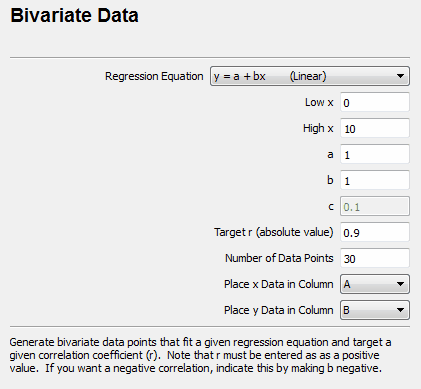﻿ Bivariate Data

# Bivariate Data

Top  Previous  NextThe bivariate data generator allows you to quickly and easily generate bivariate data that satisfies a set of given parameters.

 • Enter the number of data points to generate.  This determines the number of “dots” on the scatter graph.
 • Enter the low and high values for x.  the stat tool will generate random x values between these two values.
 • Choose the regression equation
 • Enter the parameters for the equation (a, b and c).  The c parameter is only used for quadratic regression equations.
 • Enter the absolute value of the target correlation coefficient.  the stat tool cannot calculation correlation coefficients for quadratic regression equations but it will still take the target correlation coefficient into account while generating data. If you want to generate linear regression data with a negative correlation coefficient, make b negative.
 • Enter the columns that the x and y data will be put into.